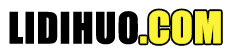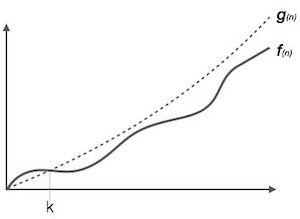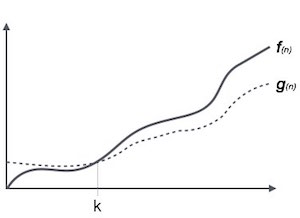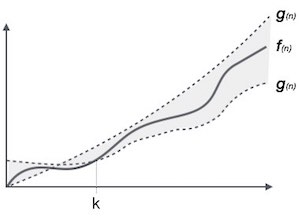# 渐近分析

Best Case-程序执行所需的最短时间。 Average Case-程序执行所需的平均时间。 Worst Case-程序执行所需的最长时间。

## 渐近符号

Ο 符号 Ω 符号 θ 符号

### O符号，Ο```Ο(f(n)) = { g(n) : there exists c > 0 and n0 such that f(n) ≤ c.g(n) for all n > n0. }
```

### Ω符号，Ω```Ω(f(n)) ≥ { g(n) : there exists c > 0 and n0 such that g(n) ≤ c.f(n) for all n > n0. }
```

### θ符号，θ```θ(f(n)) = { g(n) if and only if g(n) =  Ο(f(n)) and g(n) = Ω(f(n)) for all n > n0. }
```

## 常用渐近符号

 常数 - Ο(1) 对数 - Ο(log n) 线性 - Ο(n) n log n - Ο(n log n) 二次元 - Ο(n2) 立方 - Ο(n3) 多项式 - nΟ(1) 指数 - 2Ο(n)